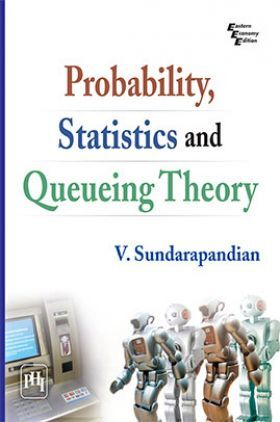# Probability, Statistics And Queuing Theory

Sold ( 80 times )
21574 Views
MRP : ₹495.00
Price : ₹470.25
You will save : ₹24.75 after 5% Discount
Inclusive of all taxes

Save extra with 2 Offers

Get ₹ 50

Instant Cashback on the purchase of ₹ 400 or above

Product Specifications

 Publisher PHI Learning All Engineering Mathematics books by PHI Learning ISBN 9788120338449 Author: Sundarapandian V Number of Pages 840 Available Available in all digital devicesAbout The Book Probability, Statistics And Queuing Theory

Book Summary:

Probability, Statistics and Queuing Theory is considered to be a tough subject by most engineering and science students all over the world. What Professor Sundarapandian with his indepth knowledge and rich and long experience strives to do is to make the concepts very clear and comprehensible to the students by his lucid presentation and illustrative approach.

The book analyses various types of random processes, spectral density functions and their applications to linear systems. Besides, it deals with the basics of queuing theory with a clear exposition of the five important queuing models. The text gives a detailed description of such topics as random variables, standard probability distribution, central limit theorem, random processes and spectral theory.

The text is profusely illustrated with examples and diagrams so as to make this rigorous subject more understandable to the students.

KEY FEATURES :

The text is comprehensive and the presentation practical.

Over 625 worked-out Examples, and over 440 Problem Sets.

Intended primarily as a text for undergraduate students of Engineering for their courses on Probability, Statistics, Random Processes and Queuing Theory, the book will also be extremely useful for undergraduate and postgraduate students of Science and postgraduate students of Engineering pursuing these courses.

CONTENTS
Preface
1 PROBABILITY
1.1 Brief History of Probability
1.2 Sample Space and Events
1.3 Classical and Empirical Probability
1.4 Axiomatic Definition of Probability
1.5 Conditional Probability
1.6 Total Probability
1.7 Bayes Theorem
2 RANDOM VARIABLE
2.1 Definition of a Random Variable
2.2 Distribution Function of a Random Variable
2.3 Discrete Random Variable
2.4 Continuous Random Variable
2.5 Mathematical Expectation
2.6 Chebyshevs Inequality
2.7 Moments of a Random Variable
2.8 Moment Generating Function
2.9 Characteristic Function
3 STANDARD PROBABILITY DISTRIBUTIONS
3.1 Degenerate Distribution
3.2 Bernoulli Distribution
3.3 Binomial Distribution
3.4 Poisson Distribution
3.5 Geometric Distribution
3.6 Negative Binomial Distribution
3.7 Uniform Distribution
3.8 Exponential Distribution
3.9 Gamma Distribution
3.10 Weibull Distribution
3.11 Normal Distribution
3.12 Functions of a Random Variable
4 TWO-DIMENSIONAL RANDOM VARIABLES
4.1 Joint Distribution Functions
4.2 Marginal Distributions
4.3 Conditional Distributions
4.4 Expectation
4.5 Sums of Independent Random Variables
4.6 Functions of Random Variables
4.7 Covariance
4.8 Conditional Expectation
4.9 Correlation and Regression
4.10 Central Limit Theorem
5 RANDOM PROCESSES
5.1 Definition and Description of Random Processes
5.2 Stationary Random Processes
5.3 Autocorrelation and Cross-correlation Functions
5.4 Ergodic Process
5.5 Markov Process
5.6 Binomial, Poisson and Normal Processes
5.7 Sine Wave Process
5.8 Birth and Death Process
6 SPECTRAL ANALYSIS OF RANDOM PROCESSES
6.1 Autocorrelation and Cross-correlation Functions
6.2 Power Spectral Density and Cross-spectral Density Functions
6.3 Linear Systems with Random Inputs
7 QUEUING THEORY
7.1 Basics of Queuing Models
7.2 Model I (M = M = 1): (/FIFO) Model, Single Server with Infinite Capacity
7.3 Model II (M = M = s): (/FIFO) Model, Multiple Server with Infinite Capacity
7.4 Model III (M = M = 1): (k/FIFO) Model, Single Server with Finite Capacity
7.5 Model IV (M = M = s): (k/FIFO) Model, Multiple Server with Finite Capacity
7.6 The (M = G = 1) Queuing System